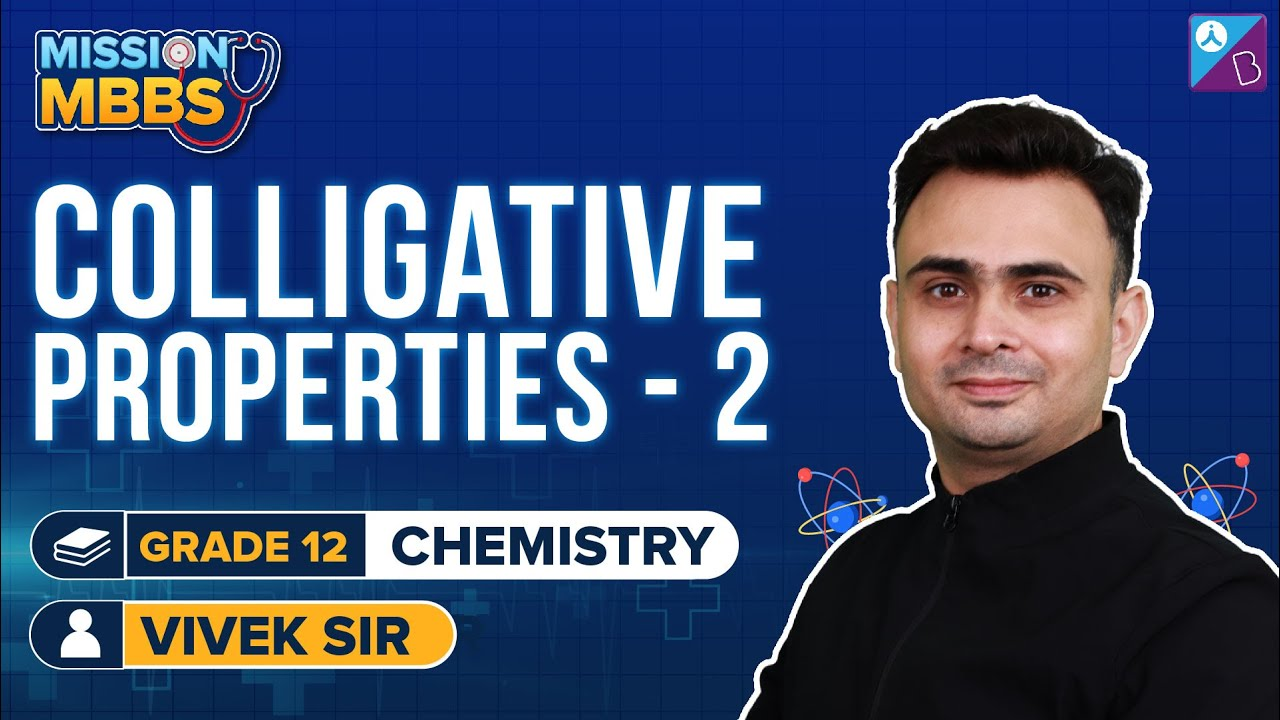# Solution and Colligative Properties Formulas for NEET

A solution is a homogeneous mixture of two or more substances on a molecular level. The constituent of the mixture present in a smaller amount is called the solute and the one present in a larger amount is called the solvent.Do Check: NEET Answer Key 2022

## Concentration of Solution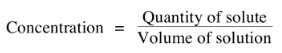## Osmotic Pressure

Osmotic Pressure is the best colligative property to determine the molecular weight of non-volatile substances.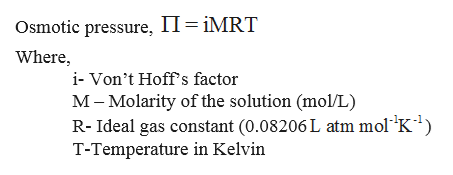## Types of Solutions

Fluids in the body generally aren’t found in pure forms. They are usually found in three types of solutions: isotonic, hypotonic and hypertonic.

• Isotonic solutions – An isotonic solution has the same solute concentration as another solution.
• Hypotonic solution – A hypotonic solution has a lower solute concentration than another solution.
• Hypertonic solution – A hypertonic solution has a higher solute concentration than another solution.

## Van’t Hoff Correction Factor

To explain the abnormal values of colligative properties and consequently molecular masses a correction factor known as Van’t Hoff Factor is employed.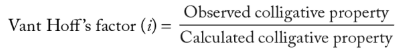## Relative Lowering of Vapour Pressure

It is the ratio of lowering of vapour pressure to vapour pressure of pure solvent.

Relative lowering of vapour pressure is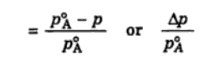## Raoult’s Law

The partial pressure of a solvent is equal to the product of mole fraction of the solvent and its vapour pressure in a pure state.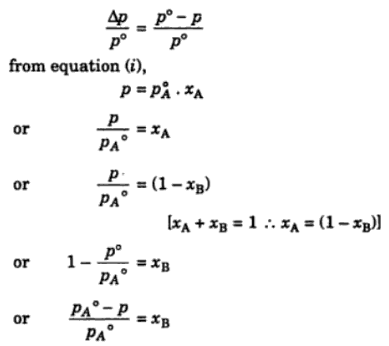## Elevation of Boiling Point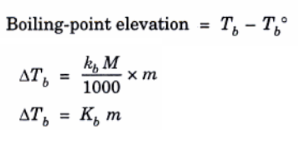## Freezing Point Depression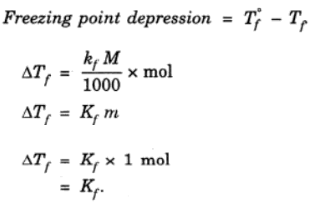## Determination of Molecular Mass of a Solute

Measurement of boiling point elevation or freezing point depression using Beckmann thermometer is a convenient method for the determination of the molecular masses of the solutes. In fact, it is a method of determining the number of moles of the solute particles present in a solution.

Mass of solute present in 1kg solvent.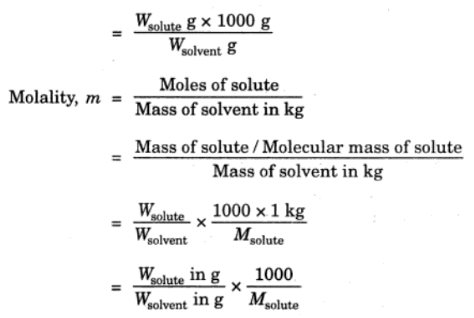Using the relation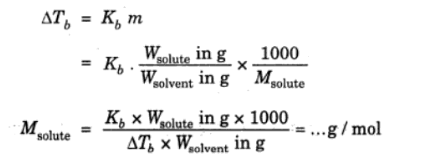Similarly, depression in freezing point is measured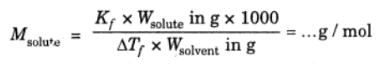## Ideal Solutions

The solutions are said to be ideal if they fulfil the following requirements.

They obey Raoult’s law

ΔHmix = 0

ΔVmix = 0

In case of ideal solutions, the vapour pressure of the solution is a linear function of mole fraction.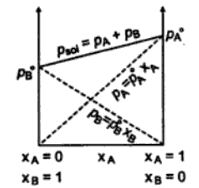## Non-ideal Solution with Positive Deviations

Non-ideal solutions exhibit positive deviations from Raoult’s law.

In such solutions.

ΔHmix > 0

ΔVmix > 0

The vapour pressure of the solution, in this case, is always larger than that expected from ideal behaviour.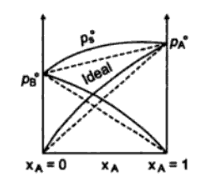## Non-ideal Solution with Negative Deviations

Non-ideal solutions exhibit negative deviations from Raoult’s law.

In such solutions.

ΔHmix < 0

ΔVmix < 0

The vapour pressure of the solution, in this case, is always smaller than that expected from ideal behaviour.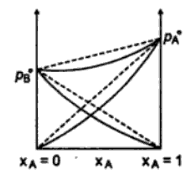## Henry Law

Henry law deals with the variation of solubility of gases in liquids with pressure. It states that solubility of gas at a given temperature is directly proportional to the pressure at which it is dissolved.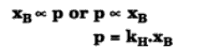This expression is identical with Raoult’s law for a volatile solute. All systems which obey Raoult’s law must satisfy Henry’s law, but the reverse will only be true if Henry’s law applies over the whole range of concentration.

Searched NEET Chemistry articles:

### Recommended Videos: